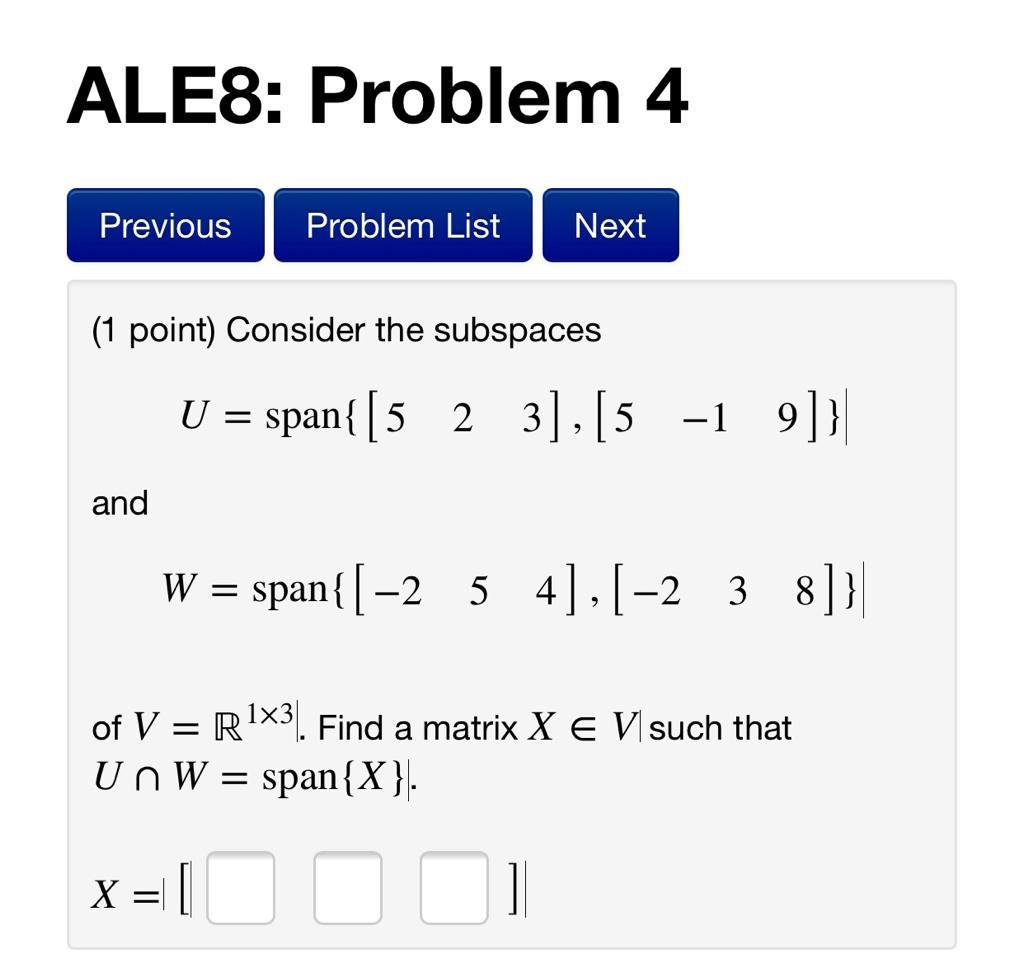### Create an Account

Already have account?

### Forgot Your Password ?

Home / Questions / ALE8: Problem 4 Previous Problem List Next (1 point) Consider the subspaces U = span{ [5 2...

# ALE8: Problem 4 Previous Problem List Next (1 point) Consider the subspaces U = span{ [5 2 3], [5 -1 9]}| and W = span{[-2. 5 4],[-23 8]}] of V = R1X3. Find a matrix X E V such that Un W = span{X}. x=

ALE8: Problem 4 Previous Problem List Next (1 point) Consider the subspaces U = span{ [5 2 3], [5 -1 9]}| and W = span{[-2. 5 4],[-23 8]}] of V = R1X3. Find a matrix X E V such that Un W = span{X}. x= [00 1Apr 15 2021 View more View Less

#### Answer (Solved)Subscribe To Get Solution